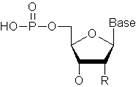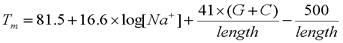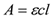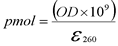Molecular Weight

The molecular weight of a substance is the weight of one mole of a that substance. To calculate the molecular weight of an oligonucleotide, the molecular weights of the individual nucleotides present in the oligo must first be summed. Then the weight must be corrected by subtracting 62.97, which is equal to two oxygen atoms and a phosphorus atom, to account for the 5’-terminal OH group, and by adding 1.008, which is equal to one hydrogen atom, to account for the 3’-terminal OH group.

The nucleotide unit:For DNA: R = H; “Base” can be Adenine, Cytosine, Guanine, or Thymine.

For RNA: R = OH; “Base” can be Adenine, Cytosine, Guanine, or Uracil.

DNA: MW = (#dA x 314.21) + (#dC x 289.18) + (#dG x 329.21) + (#dT x 304.19) – 62.97 + 1.008

RNA: MW = (#A x 330.21) + (#C x 305.18) + (#G x 345.21) + (#U x 306.17) – 62.97 + 1.008

To calculate the molecular weight of Oligo Potassium Salts, the molecular weight of the normal oligonucleotide must first be calculated (see above). This weight is then corrected by adding 39.1, which is equal to one potassium atom, and subtracting 1.008, which is equal to one hydrogen atom. This correction accounts for replacing all the acidic protons on the backbone with potassium ions.

K-Salt: MW = MW(normal) + (# nucleotides -1) x (39.1 – 1.008)
Melting Temperature:

The melting temperature of an oligonucleotide is dependent upon a number of factors including the length and G+C content of the sequence as well as the type and concentrations of cations present, particularly the sodium ion, Na+. A variety of formulas have been used for predicting melting temperatures. The following formula is recommended for oligonucleotides ranging in length from 20 to 100 residues and sodium concentrations ([Na+]) ranging from 0.01 M to 1.0 M.Extinction Coefficient:

e260, the extinction coefficient at 260 nm, is a constant that indicates the extent to which a given DNA or RNA strand in solution absorbs light at 260 nm.
In general, the calculation of the extinction coefficient of an oligonucleotide of length N can be given by the expression:e
N is the “nearest-neighbor” extinction coefficient of neighboring nucleotidesiandi + 1.
Nearest-neighbor extinction coefficients at 260 nm:

 i = C i = G i = A i = U i = dC i = dG i = dA i = dT i + 1 = C 14200 17800 21000 16200 14400 17900 21050 15200 i + 1 = G 17400 21600 25200 21200 17500 21600 25200 20000 i + 1 = A 21000 25000 27400 24000 21100 25000 27400 22800 i + 1 = U 17200 20000 24600 19600 17200 20000 24600 18200 i + 1 = dC 14400 17900 21050 16200 14600 18000 21100 15200 i + 1 = dG 175000 21600 25200 21200 17600 21600 25200 20000 i + 1 = dA 21100 25000 27400 24000 21200 25000 27400 22800 i + 1 = dT 16200 19000 23400 18200 16200 19000 23400 16800

eI is the “individual” extinction coefficient for nucleotidei.
Individual extinction coefficients at 260 nm:

 i = C i = G i = A i = U i = dC i = dG i = dA i = dT 7200 11500 15400 9900 7400 11500 15400 8700
Molarity:
Molarity is a measurement of concentration. It is equivalent to moles (mol) of solute per liter (L) of solution as expressed in the equation below.Absorbance:
The concentration of oligonucleotide in a sample is measured by its absorbance at 260 nm according to the Beer-Lambert law.where A is the absorbance (optical density or OD), ε is the molar extinction coefficient, c is the concentration, and l is the pathlength.
Annealing Temperature
To calculate the annealing temperature of oligonucleotides, first calculate the melting temperature (see above), then subtract approximately 5–10 degrees. If the two oligonucleotides have different melting temperatures do NOT average the numbers. Use the lower number so that both of the oligonucleotides can anneal. For 10mers, Eurofins Genomics recommends that PCR be done at 32–33 °C in order to maximize repeatability.
Picomoles
Picomole is a measurement of amount. The following equation can be used to calculate picomoles from the optical density (OD) and extinction coefficient (e260) of the oligonucleotide.Micrograms
Microgram is a measurement of weight. The following equation can be used to calculate micrograms from the picomoles (pmol) and molecular weight (MW) of the oligonucleotide.Note: for an oligonucleotide 1 OD260 = 33 µg.

Eurofins Genomics offers a variety of design tools to help support your needs as a researcher.  Please use the links below to use each design tool.